# Kullback-Leibler information

Kullback–Leibler quantity of information, Kullback–Leibler information quantity, directed divergence

For discrete distributions (cf. Discrete distribution) given by probability vectors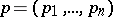,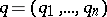, the Kullback–Leibler (quantity of) information ofwith respect tois:whereis the natural logarithm (cf. also Logarithm of a number).

More generally, one has: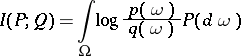for probability distributionsandwith densities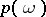and(cf. Density of a probability distribution).

The negative of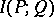is the conditional entropy (or relative entropy) ofwith respect to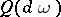; see Entropy.

Various notions of (asymmetric and symmetric) information distances are based on the Kullback–Leibler information.

The quantity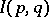is also called the informational divergence (see Huffman code).

How to Cite This Entry:
Kullback-Leibler information. Encyclopedia of Mathematics. URL: http://encyclopediaofmath.org/index.php?title=Kullback-Leibler_information&oldid=22681
This article was adapted from an original article by M. Hazewinkel (originator), which appeared in Encyclopedia of Mathematics - ISBN 1402006098. See original article